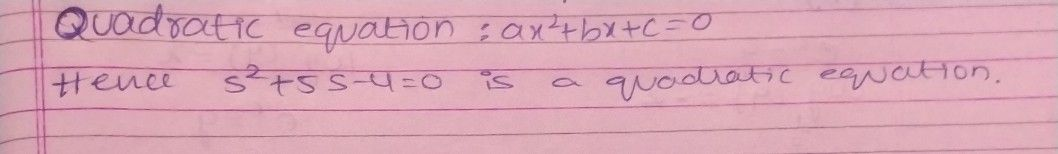Symbol
ProblemLInear equUation b. linear inequality c. quadratic equation d. quadratic Inequality $2.$ Which of the following is a quadratic equation? a. $2r2+4r-1$ b. $3t-7=2$ C. $5^{2+5s-4}=0$ $d$ $22-7x\geq 3$ $3$ in the quadratic eguation $3x2+7x-4=0$ which is the $14c-4x$ $4=$ term?
7th-9th grade
Algebra
Search count: 118
SolutionQanda teacher - RajiStudent
thank u